# Artem's blog

Mainly .NET (C#, ASP.NET) and my projects

# Release notes for SKGL Extension (2.0.5)

Development of our new Web API is going quite fast. Therefore, it is important to keep SKGL Extension up-to-date so that you can take an advantage of all new features! Today, I want to mention some of the changes that were added to SKGL Extension since 2.0.4.

### Changes

• Get Activated Machines is a new method in the Web API (see here) that allows you to get all the activated devices (machine code, IP and Time) for any given key. Note, you need to explicitly enable it as well as use your privateKey for each request. This method has the same authentication level as key generation. An example is shown below:

As you can see, in contrast to the classical operations such as validation and activation, pid, uid and hsum are passed in as a JSON array. I hope that all methods will work that way as soon as possible, because it is very easy to simply copy and paste from here and click on “Get C# friendly version”.
• Load and Save Key Information to File will from now on take care of all error handling. This means that you need not to surround these method calls with a try-catch-finally. It’s great because you don’t need to worry if the stream is open during an exception.

# Release notes for SKGL Extension (2.0.4)

I am happy to tell all users of SKGL Extension that there is a new version available (since the update in late January). In this post, I would like to mention some changes made to the API, a short usage tip, and then let you know how it is going to change in future.

### Changes

• Ability to sign pid, uid, activation dateBased on user feedback, the Web API now allows pid, uid and hsum to be added into the signature. This is particularly important for users that protect more than one product. Before, it was possible to use the same KeyInformation file (serialized by SaveKeyInformationToFile) to unlock other products (that used the same Public Key, i.e. by the same software vendor). For some, it worked out by specifying this information in the Notes field. This change makes it more simple. The activation date is intended for offline key validation; it’s a way to keep track the date of the last activation in order to force the user to activate the software periodically.
• Deactivation: There is a new method that allows you to deactivate a license key. (method info, web api)
• Optional Field: Finally you can access the optional field using .NET.  It’s a new type of method that takes in a ProductVariables object instead of separate strings for pid, uid, hsum, etc. Read more below Future ideas  (more info).
• Machine code fix: The getEightDigitsLongHash was modified to fix a bug that generated nine digits instead of eight. Read more here.
• Proxy: Get Parameters method allows you to specify the proxy settings.
• Load Product Variables: A new method, LoadProductVariablesFromString allows you to load a serialized version of the ProductVariables object. Read more below Future ideas.

### Usage tip

Some users have encountered problems when validating/activating keys because of a wrong code snippet (NullReferenceException). Basically, you should not check the .IsValid field as way to deduce whether the key is valid or invalid. Instead, check if the KeyInformation object is null.

### Future ideas

As new functionality is added to the Web API,  more parameters have to be added to each method. In the long run, this is bad because it can be quite repetitive/confusing to add all the parameters all over again. My plan on how this can be resolved is by adding specialized classes, such as ProductVariables to facilitate reuse of existing variables in other methods. The goal is to make it as easy as possible to use SKGL Extension.

# What’s new in SKGL Extension for .NET (v. 2)

The extension API for SKGL used to communicate with the Web API of Serial Key Manager has now been upgraded to support Web API 2.0. Below, I’ve listed some changes:

• The project was moved to GitHub: You can find it here.
• Some methods were removed/renamed: From now on, there is only one method for a specific action (eg. Activate). There is, for instance, only one method to perform activation, and that method will return a KeyInformation object rather than just stating whether the key is valid or invalid.
• Support for error handling: When something is missing, you will receive an error. All errors are well documented here. In debug mode, these errors will be displayed in the output.
• New hash methods and improved algorithm for data collection: The API uses an improved version of information collection (see all contributors) that is later hashed by a hashing algorithm. There are two algorithms at the movement: one that will calculate an eight digit long hash and a second one that will use SHA1. It’s always possible to pick any other hashing algorithm.

I can imagine that some of the changes may or may not trigger different kinds of emotions, partly because some code has to be modified. However, this upgrade is necessary as it will allow much more functionality to be added in the future. It’s also easier to use and thus make it work on other platforms.

Therefore, I will be available to answer some questions on how to migrate to the new library. I can’t guarantee a fast response time, but I will do my best. To save my time, please look through this page first, before contacting me. BUT first, please consider submitting your question on https://github.com/artemlos/SKGL-Extension-for-dot-NET/issues/new. If you rather prefer CodePlex, please use https://skgl.codeplex.com/discussions/topics/5452/help-support.

# Keeping Track of Code Quality with NDepend

In the recent lecture at the Computer Science programme, we’ve discussed the way projects should be designed. There are three very important criteria: Coupling, Cohesion and Responsibility driven design. We might question the use of encapsulation, avoidance of code duplication and writing very specialized classes. Some might argue that once we’ve solved a particular task in a limited amount of time, it’s possible to move on and solve other tasks. However, based on my experience from Mathos Project mainly, I can conclude that if the code would be written that way, the project would have collapsed. The reason is, in particular in open source projects, is that it’s not as strictly decided which team writes and maintains a particular module. One developer might contribute with a specific functionality, and another with a different functionality in the same module. Then, we might get a different set of people trying to understand what they’ve done to build on top of it. Even the authors of the code might not be able to understand or “dare to modify” their own code. This phenomena can be referred to as legacy code. In conclusion, we don’t want that to happen!

For some weeks ago, I received a copy of NDepend (Developer edition and Build machine edition) from Patrick Smacchia, the Lead Developer of NDepend. This made me very happy and I started to test it on my current projects. In this article, I am going to explore some of the functionality available in NDepend. Please note that I am just describing features that came first in to my sight. NDepend is a very advanced system and contains a lot more functionality than I’ve mentioned here. Many specialized books have referred to the system and it’s also recommended by for instance Jeffrey Richter (I’ve referred to one of his works in this article) and Scott Hanselman. (For a more complete tutorial, please see this Pluralsight course)

First impression

Once I installed NDepend and test-launched it, I was able to generate a comprehensive report without reading any documentation. It’s a friendly GUI that suggests you what you can do at any point during the code analysis.

Even if it might appear as if it’s so much new information that has to be interpreted, there is a Get Started feature built-in to the report (top, right corner). It suggests that we should look out for unwanted dependencies, look for complex methods, etc. Personally, the first thing that fell into my sight is the percentage of comments in the project. Recently, Mathos Project promoted the idea to write xml comments to each new method as it is implemented. Since all analysis are saved regularly, I could have generated a report before the first post about the xml comment idea and compared it to the recent report. The percentage would tell me if we are moving the right direction. This is good not only from a developer point of view, but a also from a project manager stand point.

Visualization of projects

It’s always good to quantify big things into something that can easily be interpreted. NDepend provides you with many kinds of graph by default, but it is also possible to specify your own parameters. Let’s look at the simplest Component Dependency graph below:

Visual Studio Ultimate allows you to generate similar graphs, but in NDepend more information is encoded into the  graph. For instance, the number of dependencies is proportional to the size of a project in the diagram. If you open this diagram in NDepend, you will be able to get more information by hovering a box. An example is show below

This is quite good if you want to spot strongly coupled classes. The thicker arrow, the more depended is a class on another class. Moreover, if a more complex analysis should be performed, we can look at code metrics. I am going to analyse complexity in terms of lines of code, but this can adjusted.

Each of these small rectangles represents a method, where the area is proportional to the lines of code that method (we can change this to another property). The rectangles selected in blue are top ten methods that either have too many variables, parameters, or lines of code (read more here). If you spot a method that you want to inspect, simply double click on it and you will be redirected directly into your project where the method is located. This is quite good if we want to spot high or low cohesion. There is a tendency that the simpler a method/class is, the more specialized is the class at a particular task.

Abstractions

In addition to the diagrams I’ve already shown above, one that I really liked (partly because of the simplicity) is the Abstractness vs. Instability graphs. I’ve compared one for Mathos Core Library and Mathos Parser.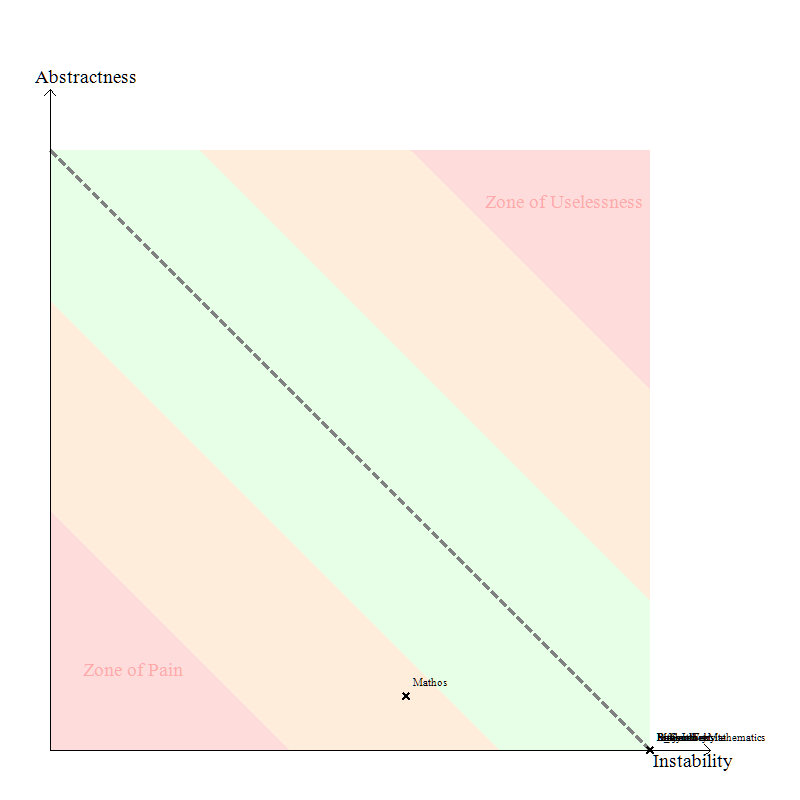Mathos Core LibraryMathos Parser

Note, Instability is a good thing since Stable means “painful to modify” according to the definition. It seems like Mathos Parser is in the safe zone and Mathos Core Library is close to it.

Creating custom rules

The report I’ve generated used the standard rules (you can see some of them here). It is possible to use the C# Linq to make your own rules. You can find more about how to construct your own rules here. It is quite straight forward. Here is one of the standard rules that measures method complexity:

An example of this functionality is shown below:Conclusion

Based on my experience with NDepend, I strongly recommend this system to developers working on projects, particularly large-scale projects. Even if you are not using .NET, I believe it’s a good idea to get some basic knowledge of it. Some weeks ago, the .NET team at Microsoft announced that the .NET Core is going to be open source, which in my opinion will make the .NET framework even more influential in environments like Linux, Mac etc. On your .NET journey, I am quite convinced that NDepend will help you to make good design decisions easier and thus make your project not only good at performing tasks, but also a pleasure to read for new developers!

Edits:

• Published 2014.012.05
• Added a picture of C# Linq overview.

# The Power of Finite Differences in Real World Problems

## Introduction

Most of the people are certainly familiar with Calculus already from high school and the beginning of university. It is usually associated with concepts like infinitely smallbehaviour at infinity, finding the area under the graph, and finding the rate of change. It seems to be so much about infinity (which is difficult to imagine) but not that much about finite values. So why not look at finite differences instead? In this article, we are going to see how powerful the concept difference is, and how we can apply it to real world problems. First, we are going to look at how the difference can be found in a sequence. Secondly, the way we can find the next term and later on how we can get a formula to generate the nth term. Finally, we are going to use the idea of finding the nth term to find an expression of the sum.

## The difference operation

It is quite intuitive, once we see a sequence, to try to find the difference between adjacent terms. If we are not satisfied, we look at the difference of the differences, and so on. Our aim now is to construct a method that will behave like we normally would in this situation. First, however, let’s look at what happens when we take the difference of each term.

 $$a_0$$ $$a_1-a_0$$ $$a_1$$ $$a_2-2a_1+a_0$$ $$a_2-a_1$$ $$a_3-3a_2+3a_1-a_0$$ $$a_2$$ $$a_3-2a_2+a_1$$ $$a_3-a_2$$ $$a_3$$

We can take the difference as many times as we like, but given that a sequence was constructed with only addition, subtraction, multiplication and division i.e. it was a polynomial, there is going to be a point when we can stop taking the difference. The important thing now to realize from this figure is that the difference expression contains the coefficients from the Pascal’s triangle. This is good if we want to take the difference many number of times. The code below utilizes this observation.

## Finding the next term

Before we proceed in finding the next term and ultimately the polynomial describing the nth term, let’s focus our attention on a real world example. Say we have the sequence $$\{n^2\}$$ i.e. $$1, 4, 9, 16 \dots$$. We assume that we don’t know the nth term, i.e. we only know some values of the sequence. The common procedure in this case is to look at the differences, similar to the previous section. For example,

 $$1$$ $$3$$ $$4$$ $$2$$ $$5$$ $$0$$ $$9$$ $$2$$ $$7$$ $$16$$

To be sure that we’ve found a pattern, we should end up at zero (assuming a polynomial generated the sequence). The interesting thing about this figure is that we know that each difference in the table can be expressed in terms of the difference expressions in the previous section. So, $$3=(4)-(1)$$ and $$2 = (9)-2\times (4)+(1)$$. This idea hints us to a possible way of finding the next term in the sequence. Since we know that the second difference $$2$$ is constant, we can find an expression for the next term by some simple arithmetic. That is, $$2=a_n -2\times a_{n-1} + a_{n-2}$$.  Thus we can find the nth term given the two terms before it. This is done by the following code:

## Finding the expression for the nth term

This is a bit more tricky to find in contrast to what we’ve done so far. The nth term is very dependent on the number of times the difference operation has to be taken before we end up at zero. In the previous example, we had to take it three times to get to zero. The polynomial we got had the second degree. It turns out that if we had to take the difference $$n$$ times to get to zero, the polynomial is of the degree $$n-1$$. This is quite useful, because if we know how the polynomial looks like, the only thing we need to find are the coefficients (more in-depth tutorial).

In the sequence $$1,4,9,16\dots$$, we had to take the difference three times, so the polynomial has the second degree, i.e. $$ax^2+bx+c$$. We have three unknowns, so we need three data points to solve the system of equations. That is,

$$\left[ {\begin{array}{*{20}{c}} 1 & 1 & 1 & 1 \\ 4 & 2 & 1 & 4 \\ 9 & 3 & 1 & 9 \end{array}} \right]$$

When we reduce this matrix to echelon form, we get that the coefficient for $$n^2$$ is $$1$$, and zero for the remaining terms. The code below does this task.

The coefficients we get correspond to a term in the polynomial. For example, if we get $$3, 2, 1$$, the polynomial is $$3x^2+2x+1$$.

## Finding the closed form for the sum

When we first think about how we can find the closed form for a sum, we might conclude that it is difficult. In Concrete Mathematics – A Foundation for Computer Science, an entire chapter (chp. 2) and a great part of other chapters is dedicated to sums, and the way we can find the anti-difference. At least, that was my thought when I got this idea. But later, an interesting thought came up to my mind, and that is to treat the sum as a sequence and the sequence as the difference of the sums’ terms. Let’s clarify this. If we have the sequence $$1,2,3,4,\dots$$, we can construct the partial sums, i.e. $$1, 1+2, 1+3+4, 1+2+3+4$$, thus $$1, 3, 6, 10$$. But the partial sums form a new sequence which we can analyse in a similar way. This means that can we can reuse quite a lot of code. Now, since in the original sequence $$1,2,3,4,\dots$$, we have to take the difference twice to get zero, in the new sequence $$1, 3, 6, 10$$, we have to take the difference three times. The code for doing this is shorter, in contrast to the previous ones.

## Limitations

One of the limitations of this code/algorithm is that we can only use sequences that have been generated by a polynomial. Exponents are not allowed, although $$n^2= n\times n$$, so that works. The reason is quite simple. Formally, we define difference as an operator on a function (similar to derivatives) as

$$\Delta f(x) = f(x+1) – f(x)$$. Let’s say we have $$f(x)=2^x$$. If we take $$\Delta(2^x)=2^{x+1}-2^x=2^x(2-1)=2^x$$. Not good, no matter how many times we take the difference, we end up with the same thing we had in the beginning. This is why this particular algorithm does not work in this case. It is possible to add an extra step that checks against known sequences each time a difference is taken. This, I will do a bit later! 🙂

## Source code

You can find all additional methods in the FiniteCalculus class in Mathos.Calculus (Mathos Core Library). Also, you can see this code in action here!

# Mathos Parser is now an expression compiler

The requirement of performing several thousands of calculations (for example, during integration) led to the optimization with pre-scanned expressions. But the aim to make Mathos Parser even faster still remained. Now, I would like to introduce you to a new project of Mathos Project – Mathos Expression Compiler, based on the math tokenizer and parser logic of Mathos Parser.

This API allows you to parse an expression and “store” it as CIL code. Then, using ILAsm, you can generate a new assembly that will contain your expression entirely parsed. The only operation that occurs at runtime is the insertion of values into the variables that you declared and the operation on that variable value. To make it even faster, most of the calculations are already done before the runtime, for example, x+3+5 would be stored as x+8.

In this scenario, the time consideration can be important. Even if it is faster to execute CIL instructions, the compilation process can take some time. This has not been tested yet. The process is illustrated below: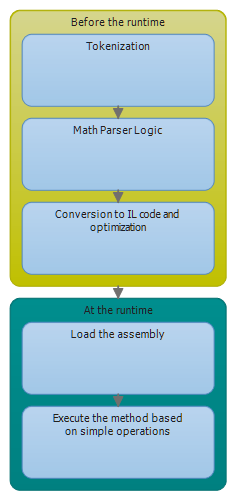This is a very simplified way of looking at it but the point is to always test Mathos Parser together with Mathos Expression Compiler and make an estimate of how much time would be saved in different scenarios. It all depends on the context. But note, that

A performance hit is incurred only the first time the method is called. All subsequent calls to the method execute at the full speed of the native code because verification and compilation to native code don’t need to be performed again. //p. 12. Jeffrey Richter. CLR via C# 3rd Edition

So, if you plan to execute the function trillion times, it might be a good a idea to keep in mind the Expression Compiler as a better alternative, because at that point, the compilation time will be negligible.

Now, back to the features. It was mentioned in the beginning that it is based on the Mathos Parser. Note, in terms of features, Mathos Expression Compiler has at this point only a subset of those of Mathos Parser. At this point, you cannot have functions as sincos, and your own custom functions, but this will be changed in the nearest future.

There are also some changes to the way operators work. To declare an operator properly, you should use the variables OperatorListOperatorAction, and OperatorActionIL. Below, a simple example of addition operator:

Comparison operators are a bit more tricky. An example of greater than operator is shown below:

For those who understand IL, the @ sign might seem a bit strange. It is simply a way to tell the stack count to the if statement (in the compilation process).

When you actually generate an expression, this is how your IL code for that expression might look:

Will return (only IL for the expression):

When you have the IL code, you should name the output executable(or dll) as MathosILParser, otherwise, you might get an error. In future, an option will be added to customize the name of the output file, but at this point, please use RegEx (or replace option) to change the MathosILParser.exe to something else (in the IL code).

Your function can later by called as shown below. Note, I am not using reflection at all.

I hope this short guide was helpful, even if it might be a bit messy. If you have performed any testing related to speed or if you want to show some examples, please feel free to comment or contact me.

# If statement in CIL

The article, Introduction to IL Assembly Languageis a great resource for those of you who would like to understand IL code (kind of like asm but in .net). IL really gives you a different perspective on computer programming, and you must consider more things in comparison to high level languages like C#.

Currently, I am studying conditional statements. Below, an example of a statement that checks whether the two values in the evaluation stack are equal to each other.

By executing this, the result below would be shown: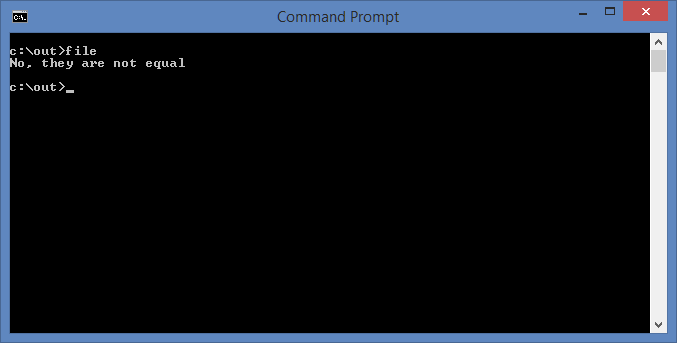# The advantages of pre-scanned expressions

RubyInt project, or Iron Ruby Based Mathos Core Library Interpreter was one of the projects to be tested with the new parser feature, that is, pre-scanned expressions.

In the Integral approximation module, it is quite good to use pre-scanned expressions, since the expression stays the same through the entire process. The execution time improved in this case.

The integral approximation website in Mathos Laboratory (see it here) has also shown good results thanks to this optimization. In Mathos Laboratory in particular, it is important that methods are quick and use as small amount of resources as possible, because it is hosted in the Cloud.

I have performed some quick tests using an i3 processor (the methods are in changeset 37964), although I would not recommend them to draw specific conclusions (there is a random error involved so more repetitions of each test are needed). Also, the difficulty level of an expression can affect the tokenization time. The graphs are located below: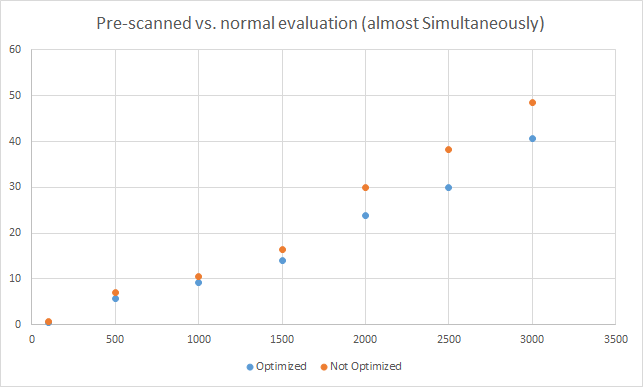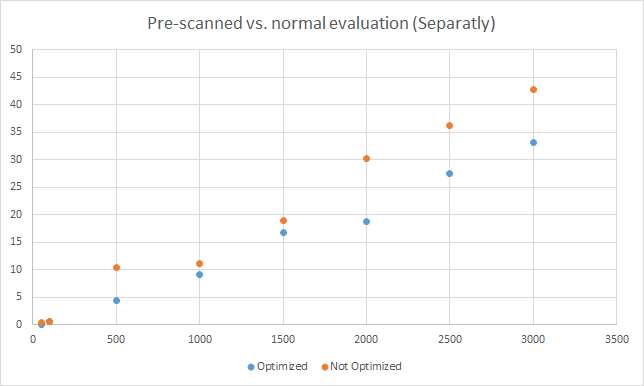One conclusion that can be drawn from this is that the optimization only gets useful when the same expression is evaluated more than 100-500 times.

# Faster evaluation using a pre-scanned expression

[This article was updated to included changes in v. 1.0.7.2. Originally written for v. 1.0.7.1]

Today, I published a newer version of Mathos Parser on both CodePlex and NuGet. The release notes:

from v.1.0.7.2:

• Force user to use ReadOnlyCollection when using a token list as a parameter.

from v. 1.0.7.1

• Changed the structure a bit. Now, the Scanner returns tokens instead of continuing with MathParserLogic. This is good if the same kind of expression is to be used many times.

But what does this really mean? Well, previous versions of the parser worked more locally, that is, once one function was executed, it triggered another function, and so on. Now, however, the Scanner (private method) returns a list of tokens instead of launching MathParserLogic directly. If you simply want to get tokens, you would have to execute the GetTokens function, as shown below:

You can then send the tokens list into either Parse or ProgrammaticallyParse and it will parse it in the same way as if you would enter a string expression, that is:

Both statements above will return 32, but there is one main difference; the execution time will be so much smaller for the second statement, where a “pre-compiled” list is being used. If it would take 100 milliseconds (ms) to find the value of the first statement, it would take approximately 9-10 ms for the second one. The ratio is 10:1.

This is great if you want to perform an operation where the same function is involved several thousands times, for example when approximating an integral.

I am currently thinking about converting math expressions into IL code, but this will happen sometime in future!

# Recorded a new video about Mathos Core Library Interpreter

Page 1 of 4:1 2 3 4 »